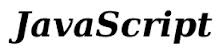﻿ How to Create a Simple Online Calculator with Javascript ﻿# How to Create a Simple Online Calculator with JavascriptIn this article, we show how to create a simple online text editor for a website with Javascript.

The calculator we will build will have functionality for the 4 most basic arithmetic operations, including addition, subtraction, multiplication, and division.

The calculator also allows for decimal numbers.

The calculator will also clear the screen after an operation is done and another is about to be performed.

The calculator cannot do more than one operation with a single calculation. Again, it's basic.

So below is the calculator.

So let's now break down the code.

Again, this calculator doesn't make many functionalities that you would find in calculators. It doesn't have a clear button, parentheses, etc.

This code serves to show how to create a basic calculator.

There is room for much improvement but this is a starter.

Let's now go over the code.

### HTML Code

So we make the calculator out of the HTML table element. This is so that we can organize the calculator buttons into nice rows and columns.

Each button is assigned to the "calculatorbuttons" class except for the equals (=) button. This button is different because it ends a calculation. You'll understand more when we go over the Javascript code.

Each button has a custom attribute, data-button, which makes us be able to differentiate one button apart from another, so that we can identify which is pressed.

A special note about this HTML code is that the area where you see the numbers and operators, the screen of the calculator, has an id tag of 'mainarea'.

Above this, we create a label element with the id of 'calculation', which will contain the original expression that a user enters. When a user clicks the '=' button, the answer replaces this original expression. This is the reason we have this label element to contain the original expression.

So this sums up the HTML code.

Next, we move to the Javascript code.

### Javascript Code

Now we go to the Javascript code.

The Javascript codes adds functionality to the calculator.

So we've broken up our Javascript code into 2 blocks.

The first block addresses what occurs after the "=" button is pressed.

So the first thing we have to do is get what the user entered in. We do this through the line, var content= \$('#mainarea').text();

To remove white space from this content, if any is present, we use the line, content= content.replace(/\s/g, "");

We then write the contents of the user input into the HTML label tag with an id of 'calculation'. This is because the contents of the screen are overwritten with the answer of the calculation. To remember what the user entered, this appears above the calculator.

Since we are creating a basic calculator that can only have 2 operands and one operator, we create a variable, firstnumber, which represents the first operand.This will match either integers or decimal numbers.

The next variable, secondnumber, represents the second operand. This, also, can be an integer or a decimal number.

Next, we have to make sure that each operand is a number so we typecast them using the Number() function.

We then create a variable, answer, which will store the result of the operation of the 2 operands.

Next we have if statements to find out which operator is being used. It can be addition, subtraction, multiplication, or division. We perform the operation based on which operator is present in the expression.

We then overwrite the screen with the answer.

The next thing that we do which is important is we create a localStorage variable. We give it the name, calculationnumber, and set its value to 1.

This will be important because an answer was calculated and is now shown on the screen. If the user now enters a new number to do a new calculation, we need to clear the contents of the screen and enter in the new expression. We need to know, though, that the "=" was clicked, so we create a localStorage variable and set it to some value, which we will use to show it was clicked. Thus, if a new number is pressed, we want to clear the contents and write in this number.

We now go to our next block of Javascript code.

The next block of code listens for button presses and will enter into the screen what button was pressed. Each button has a class of 'calculatorbuttons' except for the '=' button.

Before we go into any specific button, we want to see whether the localStorage variable is set to 1. If it is, a previous calculation was done, so we need to clear the contents of the screen. We then set the localStorage variable to 0.

To be able to know which button is pressed, we supplied each button with a data-button attribute. This attribute differentiates each button and is unique to each button. This is what the series of if statements does.

And this is a basic calculator created with Javascript.

Of course, it can be improved drastically.